# [原创] 推荐一款编写数学公式的国产神器：AxMath，可与LATEX双向转换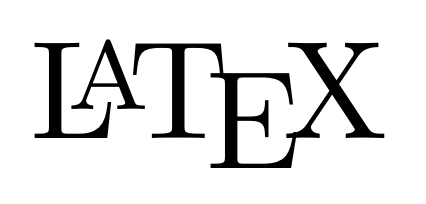\left( \sum_{i=1}^n{a_ib_i} \right) ^2\leqslant \left( \sum_{i=1}^n{a_{i}^{2}} \right) \left( \sum_{i=1}^n{b_{i}^{2}} \right) , a_i,b_i\in \mathbb{R}MathType的方便之处
MathType可以直接在复制图形界面的公式的时候，就把公式自动转换成LATEX语法再复制到内存里，粘贴的时候直接粘贴出来LATEX。比如我的网站里面的数学公式，在后台的文字编辑界面里大部分都是LATEX，但它们并不是我用手输的，而是用软件转换成LATEX再粘贴上来的（参考这个链接）。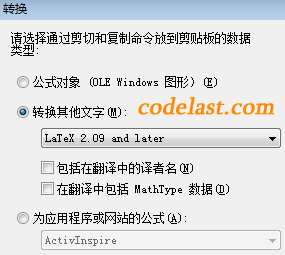MathType的缺点

MathType官方的雷人开发效率

MathType有什么替代软件吗？AxMath的局限性

AxMath的华丽功能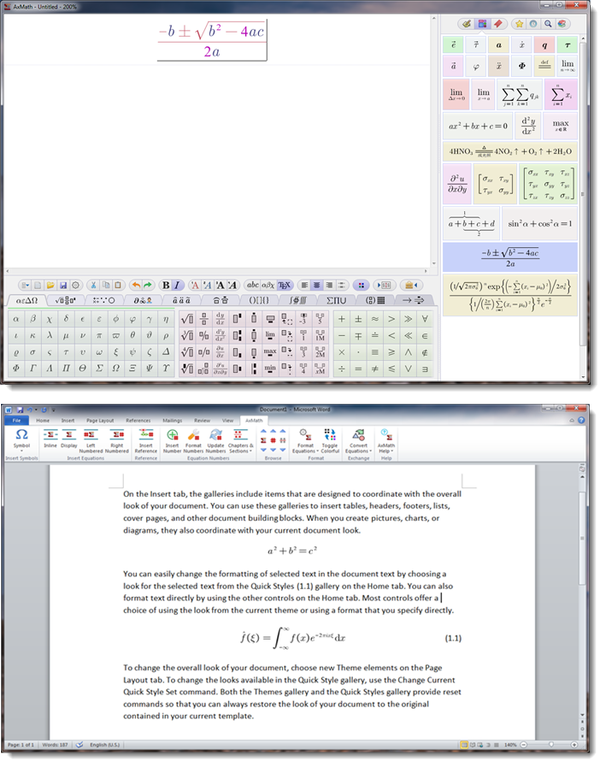可以自定义复制出来的LATEX的起始、结束符号

\ [y=ax+b\ ]

AxMath就没有这种问题，因为它可以设置“语法定界符”，也就是上面说的LATEX开头、结尾的符号。设置成 \ ( 和 \ ) 就解决问题啦！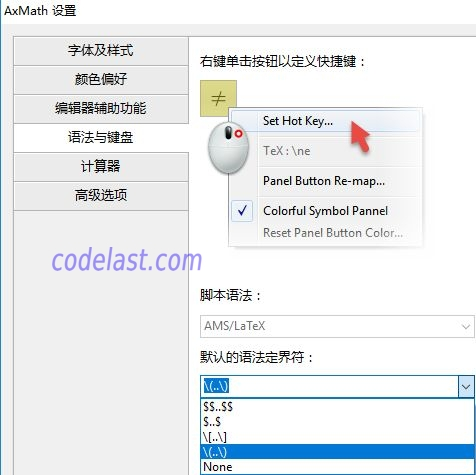没有MathType经常“多生成一个空格”的问题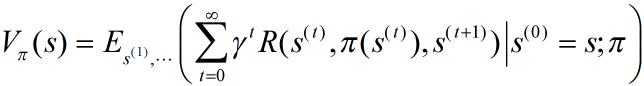\ [{V_\pi }(s) = {E_{{s^{(1)}}, \cdots }}\left( {\sum\limits_{t = 0}^\infty  {{\gamma ^t}R({s^{(t)}},\pi ({s^{(t)}}),{s^{(t + 1)}})\left| {{s^{(0)}} = s;\pi } \right.} } \right) \ ]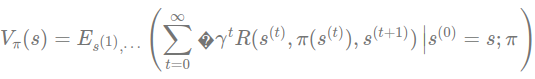结语
AxMath，你值得拥有！

➤➤ 版权声明 ➤➤